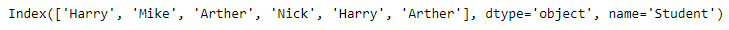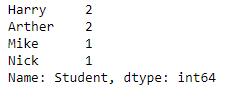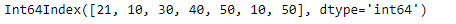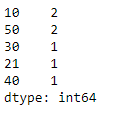# Python | Pandas Index.value_counts()

Python is a great language for doing data analysis, primarily because of the fantastic ecosystem of data-centric python packages. Pandas is one of those packages and makes importing and analyzing data much easier.

Pandas` Index.value_counts()` function returns object containing counts of unique values. The resulting object will be in descending order so that the first element is the most frequently-occurring element. Excludes NA values by default.

Syntax: Index.value_counts(normalize=False, sort=True, ascending=False, bins=None, dropna=True)

Parameters :
normalize : If True then the object returned will contain the relative frequencies of the unique values.
sort : Sort by values
ascending : Sort in ascending order
bins : Rather than count values, group them into half-open bins, a convenience for pd.cut, only works with numeric data
dropna : Don’t include counts of NaN.

Returns : counts : Series

Example #1: Use `Index.value_counts()` function to count the number of unique values in the given Index.

 `# importing pandas as pd ` `import` `pandas as pd ` ` `  `# Creating the index ` `idx ``=` `pd.Index([``'Harry'``, ``'Mike'``, ``'Arther'``, ``'Nick'``, ` `                ``'Harry'``, ``'Arther'``], name ``=``'Student'``) ` ` `  `# Print the Index ` `print``(idx) `

Output :Let’s find the count of all unique values in the index.

 `# find the count of unique values in the index ` `idx.value_counts() `

Output :The function has returned the count of all unique values in the given index. Notice the object returned by the function contains the occurrence of the values in descending order.

Example #2: Use `Index.value_counts()` function to find the count of all unique values in the given index.

 `# importing pandas as pd ` `import` `pandas as pd ` ` `  `# Creating the index ` `idx ``=` `pd.Index([``21``, ``10``, ``30``, ``40``, ``50``, ``10``, ``50``]) ` ` `  `# Print the Index ` `print``(idx) `

Output :Let’s count the occurrence of all the unique values in the Index.

 `# for finding the count of all  ` `# unique values in the index. ` `idx.value_counts() `

Output :The function has returned the count of all unique values in the index.

My Personal Notes arrow_drop_upCheck out this Author's contributed articles.

If you like GeeksforGeeks and would like to contribute, you can also write an article using contribute.geeksforgeeks.org or mail your article to contribute@geeksforgeeks.org. See your article appearing on the GeeksforGeeks main page and help other Geeks.

Please Improve this article if you find anything incorrect by clicking on the "Improve Article" button below.

Article Tags :

1

Please write to us at contribute@geeksforgeeks.org to report any issue with the above content.